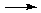Net Ionic Equations Are Important

The reason to write a chemical equation is to express what we believe is actually happening in a chemical reaction. One of the most useful applications of the concept of principal species is in writing net ionic equations. These are equations that focus on the principal substances and ions involved in a reaction--the principal species--ignoring those spectator ions that really don't get involved.

For example, consider the reaction described by the following full molecular equation:

HCl(aq) + NaOH(aq)NaCl(aq) + H2O

HCl, NaOH, and NaCl are all strong electrolytes. As such, they dissociate completely into their ions in solution, and although we might write "HCl" we really mean "H+ + Cl". Similarly, "NaOH" is "Na+ + OH" and "NaCl" is "Na+ + Cl". (For more information on classifying electrolytes, click here.)

H+(aq) + Cl(aq) + Na+(aq) + OH(aq)Na+(aq) + Cl(aq) + H2O

Notice that Na+ and Cl never really react. They are floating around at the beginning and still floating around at the end. Thus, a better equation for what is actually happening would be just:

H+(aq) + OH(aq)H2O

where we have neglected the Na+ and Cl because they are not really involved. If you want to emphasize that H+ is hydrated, then you can write:

H3O+(aq) + OH(aq)2 H2O

Writing Net Ionic Equations

Writing net ionic equtaions is easier than you might think. First of all, we MUST start with an equation that includes the physical state:

• (s) for solid,
• (l) for liquid,
• (g) for gas, and
• (aq) for aqueous solution.
The three rules for writing net ionic equations are really quite straightforward.
1. Only consider breaking up the (aq) substances.
2. Only break up strong electrolytes.
3. Delete any ions that appear on both sides of the equation.
Clearly rule 2 is the tricky one. You must know your strong electrolytes:

 strong acids HCl, HBr, HI, HNO3, HClO3, HClO4, and H2SO4 strong bases NaOH, KOH, LiOH, Ba(OH)2, and Ca(OH)2 salts NaCl, KBr, MgCl2, and many, many more, all containing metals or NH4.

This is a matter of memorizing the seven strong acids and checking for the presence of a metal or ammonium (NH4+). Note that all strong bases contain a metal, and all salts contain either a metal or ammonium.

Another Example

Here's another example:

HF(aq) + AgNO3(aq)AgF(s) + HNO3(aq)

Separating the aqueous strong electrolytes, we have:

HF(aq) + Ag+(aq) + NO3(aq)AgF(s) + H+(aq) + NO3(aq)

Note that HF is a weak acid, so we leave it together. Since AgF is a solid, we are saying that it precipitates from the reaction, and it wouldn't be right to separate it into its ions. The spectator ion in this case is NO3. It starts out in solution and ends up in solution as well, with no role in the actual reaction. We leave it out in writing the final net ionic equation:

HF(aq) + Ag+(aq)AgF(s) + H+(aq)

Again, if you want to emphasize that H+ is hydrated, then you can write:

HF(aq) + Ag+(aq) + H2OAgF(s) + H3O+(aq)

What if I don't have the products?

In some situations you only know the reactants. For example, one might need to know the net ionc equation for "the reaction between NaHSO4 and NH3." What then? There are two ways to proceed:

1. Determine the "molecular equation" and proceed as above. This works fine as long as you can figure out the product in the first place!

 NaHSO4(aq) + NH3(aq)NaNH4SO4(aq) Na+(aq) + HSO4–(aq) + NH3(aq)Na+(aq) + NH4+(aq) + SO42–(aq) HSO4–(aq) + NH3(aq)NH4+(aq) + SO42–(aq)

2. Alternatively, you can figure out what is REALLY present first and then see how they might react. This method requires just as much knowledge of electrolytes but avoids the extra step of writing the molecular equation and focuses on the chemistry:

1. NaHSO4(aq) + NH3(aq) is really a mixture of Na+(aq), HSO4(aq), and NH3(aq).
2. HSO4 is weakly acidic, while NH3(aq) is weakly basic. Thus, H+ must be transferred from the HSO4 to the NH3.
3. HSO4(aq) + NH3(aq)NH4+(aq) + SO42–(aq)